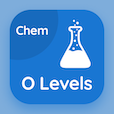Cambridge Online Courses (GCSE)

O Level Physics MCQs

O Level Physics MCQ PDF - Topics

# Measuring Temperature MCQ Quiz Online

Learn Measuring Temperature Multiple Choice Questions (MCQ), Measuring Temperature quiz answers PDF to study o level physics online course for o level physics classes. Measurement of Temperature Multiple Choice Questions and Answers (MCQs), Measuring Temperature quiz questions for ACT prep classes. "Measuring Temperature MCQ" PDF Book: measuring temperature, scales of temperature test prep for colleges that offer online classes.

"Every temperature measuring instrument makes use of a physical property of a substance in order to measure temperature objectively, which physical property is used by Mercury-in-glass thermometer?" MCQ PDF: measuring temperature with choices electromotive force, volume of a fixed mass of liquid, resistance of a piece of metal, and pressure of a fixed mass of gas at constant volume for ACT prep classes. Study measuring temperature quiz questions for merit scholarship test and certificate programs for ACT test.

## MCQs on Measuring Temperature Quiz

MCQ: Every temperature measuring instrument makes use of a physical property of a substance in order to measure temperature objectively, which physical property is used by Mercury-in-glass thermometer?

Electromotive force
Volume of a fixed mass of liquid
resistance of a piece of metal
Pressure of a fixed mass of gas at constant volume

MCQ: Every temperature measuring instrument makes use of a physical property of a substance in order to measure temperature objectively, which physical property is used by Constant-volume gas thermometer?

Electromotive force
Volume of a fixed mass of liquid
resistance of a piece of metal
Pressure of a fixed mass of gas at constant volume

MCQ: Every temperature measuring instrument makes use of a physical property of a substance in order to measure temperature objectively, which physical property is used by Thermocouple?

Electromotive force
Volume of a fixed mass of liquid
resistance of a piece of metal
Pressure of a fixed mass of gas at constant volume

MCQ: Every temperature measuring instrument makes use of a physical property of a substance in order to measure temperature objectively, which physical property is used by resistance thermometer?

Electromotive force
resistance of a piece of metal
Volume of a fixed mass of liquid
Pressure of a fixed mass of gas at constant volume

### More Topics from O Level Physics Course